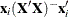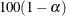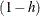# The HPREG Procedure

### OUTPUT Statement

• OUTPUT <OUT=SAS-data-set><COPYVARS=(variables)><keyword <=name>>…<keyword <=name>> ;

The OUTPUT statement creates a data set that contains observationwise statistics, which are computed after fitting the model. The variables in the input data set are not included in the output data set to avoid data duplication for large data sets; however, variables specified in the ID statement or COPYVARS= option are included.

If the input data are in distributed form, where access of data in a particular order cannot be guaranteed, the HPREG procedure copies the distribution or partition key to the output data set so that its contents can be joined with the input data.

The output statistics are computed based on the parameter estimates for the selected model.

You can specify the following syntax elements in the OUTPUT statement:

OUT=SAS-data-set
DATA=SAS-data-set

specifies the name of the output data set. If the OUT= (or DATA=) option is omitted, the procedure uses the DATAn convention to name the output data set.

COPYVAR=variable
COPYVARS=(variables)

transfers one or more variables from the input data set to the output data set. Variables named in an ID statement are also copied from the input data set to the output data set.

keyword <=name>

specifies the statistics to include in the output data set and optionally names the new variables that contain the statistics. Specify a keyword for each desired statistic (see the following list of keywords), followed optionally by an equal sign and a variable to contain the statistic.

If you specify keyword=name, the new variable that contains the requested statistic has the specified name. If you omit the optional =name after a keyword, then a default name is used.

The following are valid values for keyword to request statistics that are available with all selection methods:

PREDICTED
PRED
P

requests predicted values for the response variable. The default name is Pred.

RESIDUAL
RESID
R

requests the residual, calculated as ACTUAL–PREDICTED. The default name is Residual.

ROLE

requests a numeric variable that indicates the role played by each observation in fitting the model. The default name is _ROLE_. For each observation the interpretation of this variable is shown in Table 60.3:

Table 60.3: Role Interpretation

Value

Observation Role

0

Not used

1

Training

2

Validation

3

Testing

If you do not partition the input data by using a PARTITION statement, then the role variable value is 1 for observations used in fitting the model, and 0 for observations that have at least one missing or invalid value for the response, regressors, frequency or weight variables.

In addition to the preceding statistics, you can also use the keywords listed in Table 60.4 in the OUTPUT statement to obtain additional statistics. These statistics are not available if you use METHOD=LAR or METHOD=LASSO in the SELECTION statement, unless you also specify the LSCOEFFS option. See the section Diagnostic Statistics for computational formulas. All the statistics available in the OUTPUT statement are conditional on the selected model and do not take into account the variability introduced by doing model selection.

Table 60.4: Keywords for OUTPUT Statement

Keyword

Description

COOKD

Cook’s D influence statistic

COVRATIO

Standard influence of observation on covariance of betas

DFFIT

Standard influence of observation on predicted value

H

Leverage,LCL

Lower bound of a% confidence interval for an
individual prediction. This includes the variance of the
error, as well as the variance of the parameter estimates.

LCLM

Lower bound of a% confidence interval for the
expected value (mean) of the dependent variable

PRESS

ith residual divided by, where h is the leverage,
and where the model has been refit without the ith
observation

RSTUDENT

A studentized residual with the current observation deleted

STDI

Standard error of the individual predicted value

STDP

Standard error of the mean predicted value

STDR

Standard error of the residual

STUDENT

Studentized residuals, which are the residuals divided by their
standard errors

UCL

Upper bound of a% confidence interval for an
individual prediction

UCLM

Upper bound of a% confidence interval for the
expected value (mean) of the dependent variable